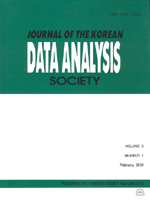상세검색
최근 검색어 전체 삭제
다국어입력
즐겨찾기0KCI등재 학술저널

# Estimation of Odds Ratio Function Used on Survival-Time Data

• 등재여부 : KCI등재
• 2002.06
• 143 - 154 (12 pages)
• 5

Epidemiological data for case-control or cohort studies are often summarized into 2x2 tables and the odds ratio is used for assessing the disease-exposure association. In this paper we define the odds ratio function(ORF) that extends odds ratio used on discrete survival-event data to continuous survival-time data, and propose estimation procedures with censored data. The first one is a nonparametric estimator based on the Nelson-Aalen estimator of cumulative hazard function, and the others are obtained using the concept of the empirical odds ratio. Asymptotic properties such as consistency and weak convergence results are also provided. The ORF provides a simple interpretation and is comparable to survival function or cumulative hazard function in comparing two groups. The mean square errors are investigated via Monte Carlo simulation. The result are finally illustrated using the Melanoma data.

1. Introduction

2. Estimation of the Odds Ratio Function

3. Asymptotic properties

4. Monte Carlo Simulation

5. Malignant Melanoma Example

References